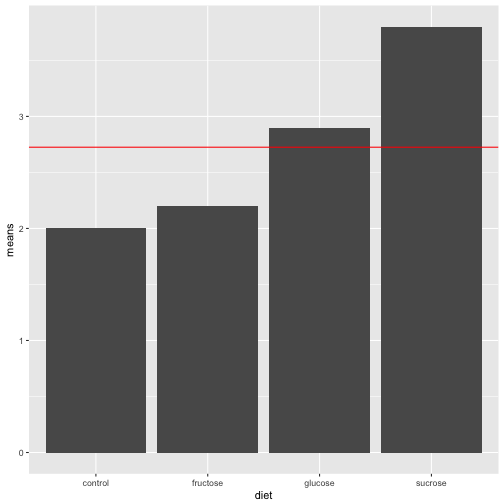# Basic Factorial Design, BF

Prof Randi Garcia
February 21, 2018

1. Explain the design prinicipal of randomization.
2. How does randomization convert variability due to differences in the experimental material into chance-like error?
3. If you have time, write any questions you have from the reading/ideas that are unclear.

### Announcements

• Project proposal due on Monday
• HW 4, due 1 week from today
• Start it now!
• Some CH 4 questions

### Agenda

• Group Time
• Analysis of variance (ANOVA)
• SS, MS, df, F
• ANOVA for the BF Design

### Leafhoppers

An experiment was conducted to compare the effects of four sugar diets on the survival of leafhoppers. The four diets were glucose and fructose (6-carbon atoms), sucrose (12-carbon), and a control (2% agar). The experimenter prepared two dishes with each diet, divided the leafhoppers into eight groups of equal size, and then randomly assigned them to dishes. Then she counted the number of days until half the insects had died.

• One factor: Diet.
• Diet has four levels: control, glucose, fructose, and sucrose.
• The response variable is the time in days that it took for half the group to die.
• The experimental unit is the group of leafhoppers.
• This is a BF design

### Leafhoppers Datalevels control sucrose glucose fructose
2.3 3.6 3.0 2.1
1.7 4.0 2.8 2.3
means 2.0 3.8 2.9 2.2

### Analysis of Variance ANOVA

Formal ANOVA starts with the simple idea that we can compare our estimate of treatment effect variability to our estimate of chance error variability to measure how large our treatment effect is.

Variability in treatment effects = True Effect Differences + Error

Variability in residuals = Error

• If our null hypothesis is, $${H}_{0}$$: True Effect Differences $$=0$$, then what would we expect the following ratio to equal?

Variability in treatment effects/Variability in residuals

### Sum of Squares (SS)

ANOVA measures variability in treatment effects with the sum of squares (SS) divided by the number of units of unique information (df). For the BF design,

${SS}_{Treatments} = n\sum_{i=1}^{a}(\bar{y}_{i.}-\bar{y}_{..})^{2}$

${SS}_{E} = \sum_{i=1}^{a}\sum_{j=1}^{n}({y}_{ij}-\bar{y}_{i.})^{2}$

where $$n$$ is the group size, and $$a$$ is the number of treatments.

### Degrees of Freedom (df)

The df for a table equals the number of free numbers, the number of slots in the table you can fill in before the pattern of repetitions and adding to zero tell you what the remaining numbers have to be.

${df}_{Treatments}=a-1$

${df}_{E}=N-a$

### Mean Squares (MS)

The ultimate statistic we want to calculate is Variability in treatment effects/Variability in residuals.

Variability in treatment effects: ${MS}_{Treatments}=\frac{{SS}_{Treatments}}{{df}_{Treatments}}$

Variability in residuals ${MS}_{E}=\frac{{SS}_{E}}{{df}_{E}}$

### F-ratios and the F-distribution

The ratio of these two MS's is called the F ratio. The following quantity is our test statistic for the null hypothesis that there are no treatment effects.

$F = \frac{{MS}_{Treatments}}{{MS}_{E}}$

If the null hypothesis is true, then F is a random variable $$\sim F({df}_{Treatments}, {df}_{E})$$. The F-distribution.

qplot(x = rf(500, 3, 4), geom = "density")


### F-Test and the null hypothesis

${H}_{0}:{\alpha}_{1}={\alpha}_{2}={\alpha}_{3}={\alpha}_{4}$

where $${\alpha}_{i}$$ is the treatment efect for the $i$th condition.

We can find the p-value for our F calculation (test statistic) with the following code

pf(17.68, 3, 4, lower.tail = FALSE)


### Treatment effects and F-ratios for all designs

• We cannot ALWAYS use the same formula for the treatment effects. It depends on inside and outside factors.
• We do not ALWAYS divide the $${MS}_{factor}$$ by $${MS}_{E}$$. To test some effects in some designs we will need a different denominator.

### Inside-outside Factors

• One factor is inside another if each group of the first (inside) fits completely inside some group of the second (outside) factor.
• Estimated effect for a factor = Average for the factor - sum of estimated effects for all outside factors.
• The sum of estimated effects for all outside factors is called the partial fit.
• Thus, the general rule is:

$Effect = Average - Partial Fit$

### Inside-outside Factors

student animal cute scary
2 cat 5 1
5 cat 5 5
1 dog 5 1
3 dog 4 2
• Draw the factor diagram as a hierarchy of inside and outside factors.
• Use this rule to calculate effects for this example we we did for the leafhoppers.

### Start HW 4

• Ch4: B1-3, C3, D1, RE CH 3: 3-4 (data in fig 3.21), 11-13, 17-19